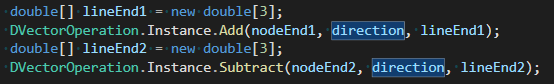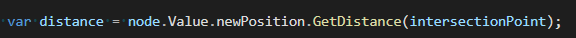# vtkCellLocator - line intersection(end point of the line)

Hi,

I’m trying to find an intersected point on a poly date with a line.
one of end points of the line is the triangle’s vertex.
I want to do not consider the triangle’s vertex as an intersected point.

As below,

So, I subtracted and added direction vector on end points.Moreover, I checked the distance between intersected point and the end point of the line.However, in some cases, it occurs end point intersection.
Is there any good solution for that?

Thank you.

Find the closest cell and Find a closest point of the poly data is the solution

1 Like